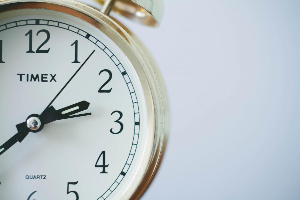# Definition of HourAn hour is a unit of time that is equal to:

• $60$ minutes
• $\dfrac{1}{24}$ days.

For example, there is one hour between $9:00\text{ am}$ and $10:00 \text{ am}$, and there are approximately $6$ hours in a school day.

### Description

The aim of this dictionary is to provide definitions to common mathematical terms. Students learn a new math skill every week at school, sometimes just before they start a new skill, if they want to look at what a specific term means, this is where this dictionary will become handy and a go-to guide for a student.

### Audience

Year 1 to Year 12 students

### Learning Objectives

Learn common math terms starting with letter H

Author: Subject Coach
You must be logged in as Student to ask a Question.